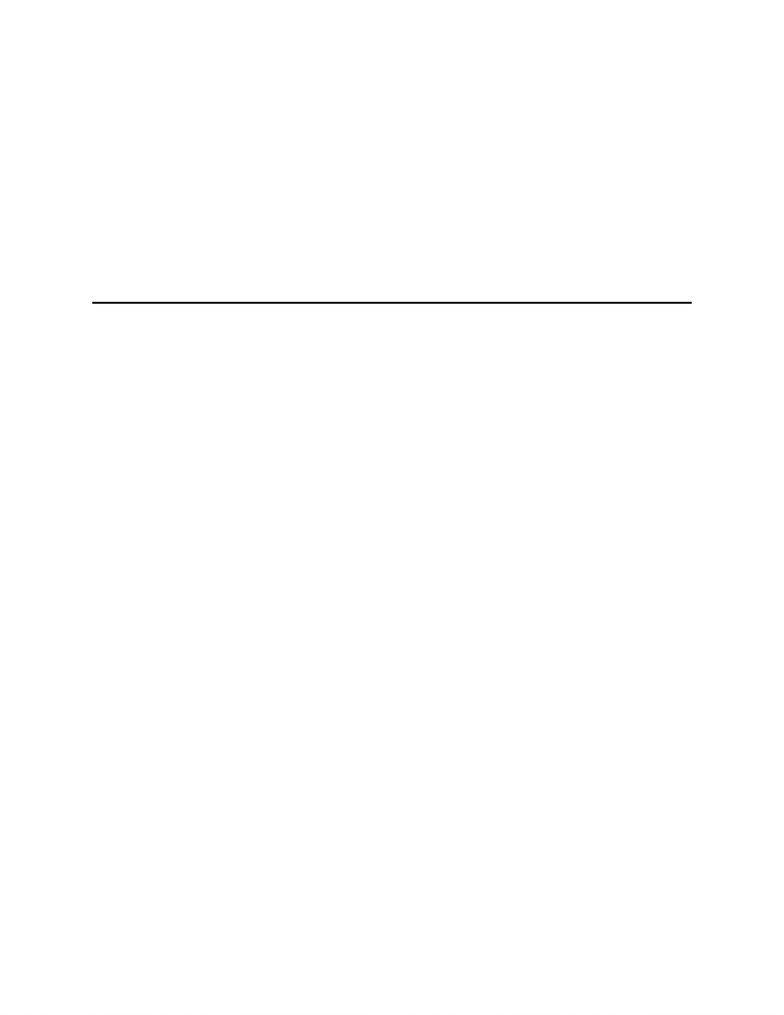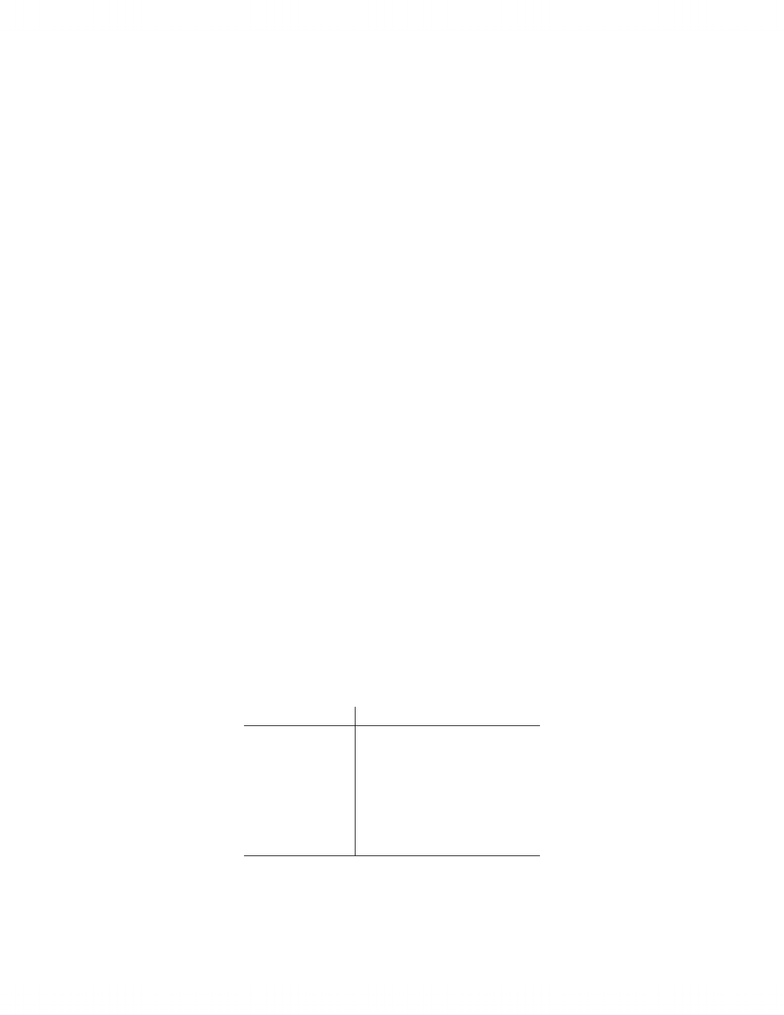# CS137 Study Guide - C Mathematical Functions, C Data Types, Scanf Format String

50 views15 pagesCS 137 - Programming Principles
Kevin Carruthers
Fall 2012
Outline
We will be covering
1. Basic C programming concepts
Variables
Integers, chars, expression evaluation
Conditionals
Loops (do, do while, for)
2. Functions, parameters, recursion
3. Arrays and pointers
4. Structures
5. Sorting, searching, time and space complexity
Absolute Basics
// import standard input/output library
#include <stdio.h>
// main function returns and integer and takes no (void) parameters
int main(void) {
// print formatted "Hello, World!"
printf("Hello, World!");
// returns 0, ie successful completion
1
Unlock document

This preview shows pages 1-3 of the document.
Unlock all 15 pages and 3 million more documents.return 0;
}
Inputs
#include <stdio.h>
int main(void) {
// declare variables
int a,b,r;
// take two integers as input, assign them to a and b. %d is for integers
scanf("%d,%d",&a,&b);
// so long as b is non-zero...
while(b) {
// r is the remainder of a divided by b
r = a % b;
a = b;
b = r;
}
printf("%d\n",a);
return 0;
}
Integer Data Types
Table 1: Types of integers and their sizes and ranges.
Type Size Range
unsigned char 1 byte [0,281]
char 1 byte [281,281]
unsigned short 2 bytes [0,216 1]
short 2 bytes [2161,216]
unsigned int 4 bytes [0,232 1]
int 4 bytes [2321,2321]
long 4/8 bytes [2641,2641
2
Unlock document

This preview shows pages 1-3 of the document.
Unlock all 15 pages and 3 million more documents.Logic
False is denoted by zero, true is denoted by non-zero (traditionally one). The logical operators
are
NOT ( ! )
OR ( || )
AND ( && )
DeMorgan’s Identities
!(P && Q) == !P || !Q
!(P || Q) == !P && !Q
Loops
while(expression)
statement // executes at least 0 times
do
statement
while(expression); // executes at least 1 time
Functions
// create function gcd which can be called with gcd(x,y) and returns an integer answer
int gcd(int a, int b) {
int r = a % b;
while(r) {
a = b;
b = r;
r = a % b;
}
return b;
}
int main() {
// print the result of a function call with arguments 806 and 338
printf("%d\n",gcd(806,338));
3
Unlock document

This preview shows pages 1-3 of the document.
Unlock all 15 pages and 3 million more documents.

## Document Summary

We will be covering: basic c programming concepts, variables, integers, chars, expression evaluation, conditionals, loops (do, do while, for, functions, parameters, recursion, arrays and pointers, structures, sorting, searching, time and space complexity. // main function returns and integer and takes no (void) parameters int main(void) { // take two integers as input, assign them to a and b. // so long as b is non-zero while(b) { // r is the remainder of a divided by b r = a % b; a = b; Table 1: types of integers and their sizes and ranges. Type size unsigned char char unsigned short short unsigned int int long. False is denoted by zero, true is denoted by non-zero (traditionally one). The logical operators are: not ( ! , or ( || , and ( && ) Demorgan"s identities (p && q) == !p || !q (p || q) == !p && !q.